Question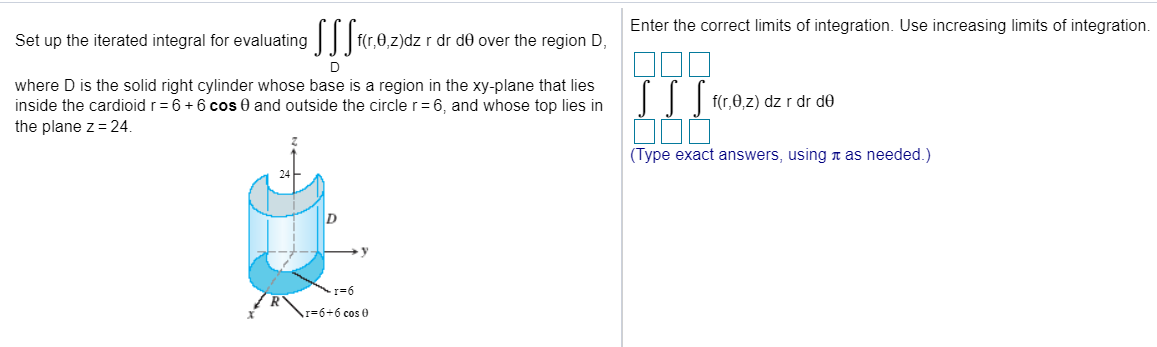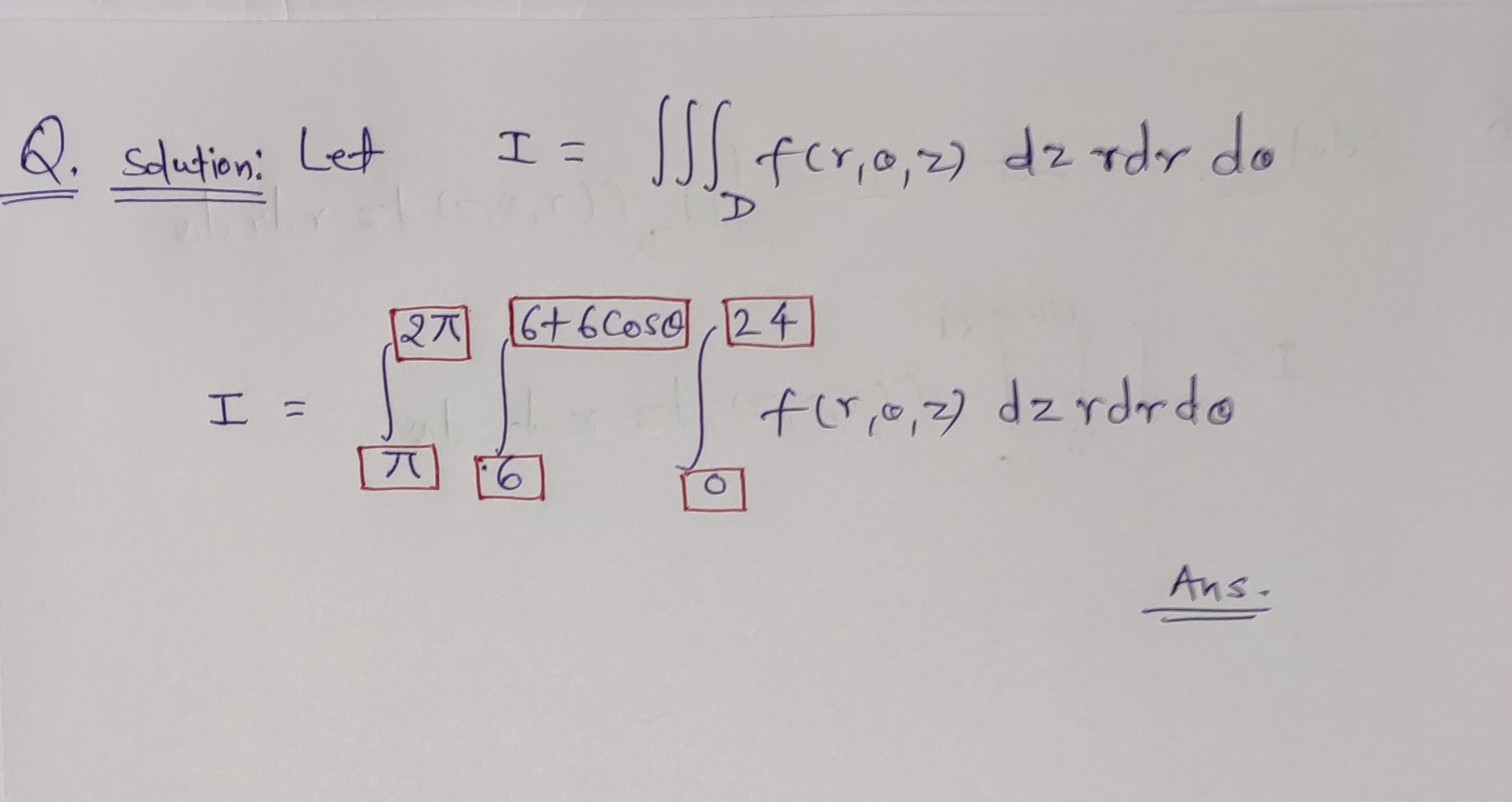#### Earn Coins

Coins can be redeemed for fabulous gifts.

Similar Homework Help Questions
• ### QUESTION 9 Set up the iterated integral for evaluating S SS Fr, 0, 2) dz r...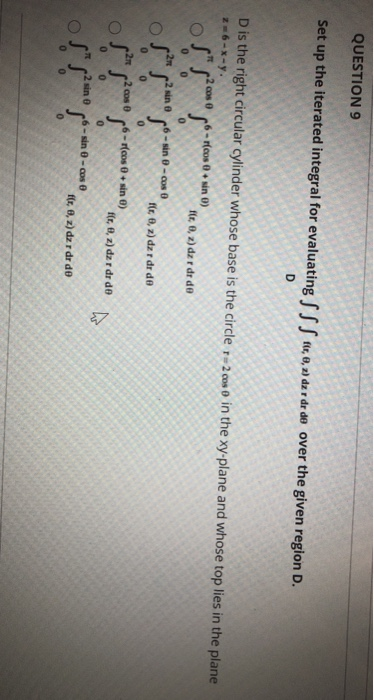QUESTION 9 Set up the iterated integral for evaluating S SS Fr, 0, 2) dz r dr de over the given region D. D D is the right circular cylinder whose base is the circle 1-2cose in the xy-plane and whose top lies in the plane 26-x-y. cos sin ) S" s2.com fit, 0, 2) dar dr de 0 sin e 6-sin-coso 52" s 'S ft , z) dar dr de so 0 0 0 0 2 cos 0 -pleos...

• ### 14.7.35 firez) dz r dr do as an iterated integral over the region that is R...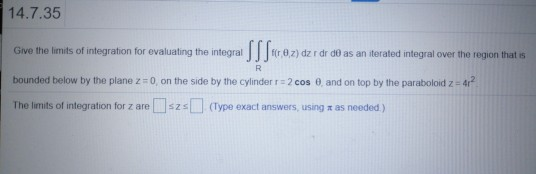14.7.35 firez) dz r dr do as an iterated integral over the region that is R Give the limits of integration for evaluating the integra SSS«.02) . bounded below by the plane z = 0 on the side by the cylinder r = 2 cos 0 and on top by the paraboloid z = 4r? IssType exact answers, using a as needed) The limits of integration for z are

• ### 5. Set up the iterated integral for evaluating SSS, f(r,0,z)dzrdrde over the given region D. D...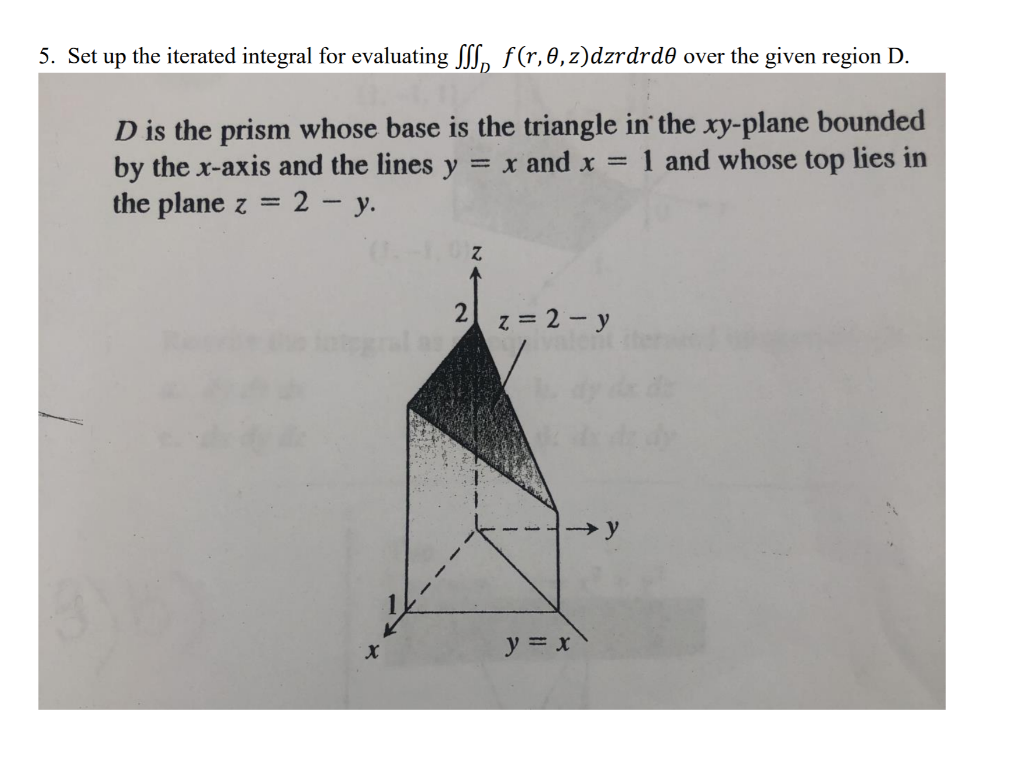5. Set up the iterated integral for evaluating SSS, f(r,0,z)dzrdrde over the given region D. D is the prism whose base is the triangle in the xy-plane bounded by the x-axis and the lines y = x and x = 1 and whose top lies in the plane z = 2 – y. z 2 z = 2 - y y = x

• ### 6. (4 pts) Consider the double integral∫R(x2+y)dA=∫10∫y−y(x2+y)dxdy+∫√21∫√2−y2−√2−y2(x2+y)dxdy.(a) Sketch the region of integration R in Figure 3.(b)...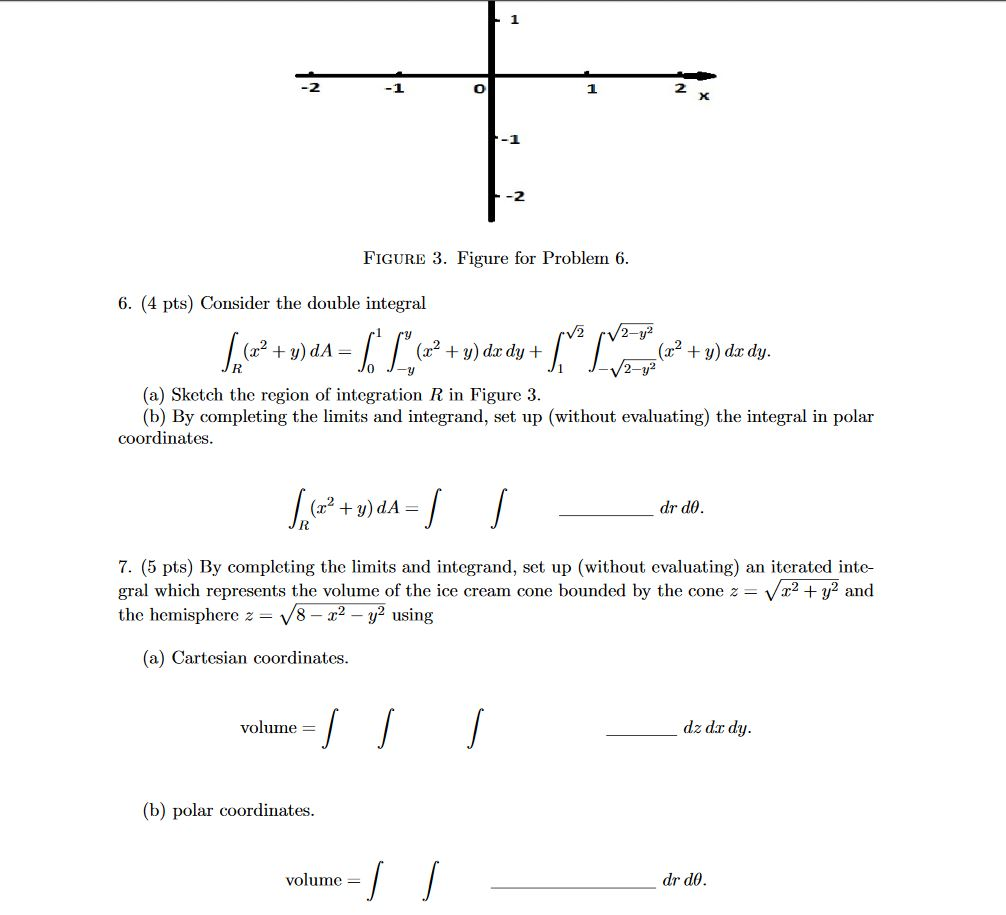6. (4 pts) Consider the double integral∫R(x2+y)dA=∫10∫y−y(x2+y)dxdy+∫√21∫√2−y2−√2−y2(x2+y)dxdy.(a) Sketch the region of integration R in Figure 3.(b) By completing the limits and integrand, set up (without evaluating) the integral in polar coordinates.∫R(x2+y)dA=∫∫drdθ.7. (5 pts) By completing the limits and integrand, set up (without evaluating) an iterated inte-gral which represents the volume of the ice cream cone bounded by the cone z=√x2+y2andthe hemisphere z=√8−x2−y2using(a) Cartesian coordinates.volume =∫∫∫dz dxdy.(b) polar coordinates.volume =∫∫drdθ. -1 -2 FIGURE 3. Figure for Problem 6. 6. (4 pts)...

• ### can someone explain the solution for this? 2. Set up an iterated integral for S. 6zdV...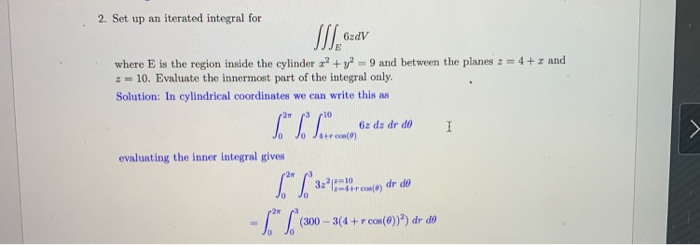can someone explain the solution for this? 2. Set up an iterated integral for S. 6zdV L" SLO པ་ཁ་ནི where is the region inside the cylinder x2 + y2 - 9 and between the planes 2 = 4 + and 2 = 10. Evaluate the innermost part of the integral only. Solution: In cylindrical coordinates we can write this as 6z dx dr de Jo Jetron) evaluating the inner integral gives 6" / 33 ir con() dr de - 6...

• ### 6. Set up, but do not evaluate, an iterated integral that gives the volume of the solid region th...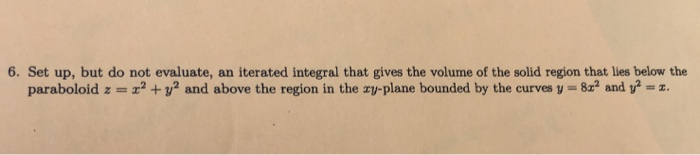6. Set up, but do not evaluate, an iterated integral that gives the volume of the solid region that lies below the paraboloid z =エ2 + V2 and above the region in the zy-plane bounded by the curves-8a2 and i-z. 6. Set up, but do not evaluate, an iterated integral that gives the volume of the solid region that lies below the paraboloid z =エ2 + V2 and above the region in the zy-plane bounded by the curves-8a2 and i-z.

• ### 7. (5 pts) By completing the limits and integrand, set up (without evaluating) an iterated inte-...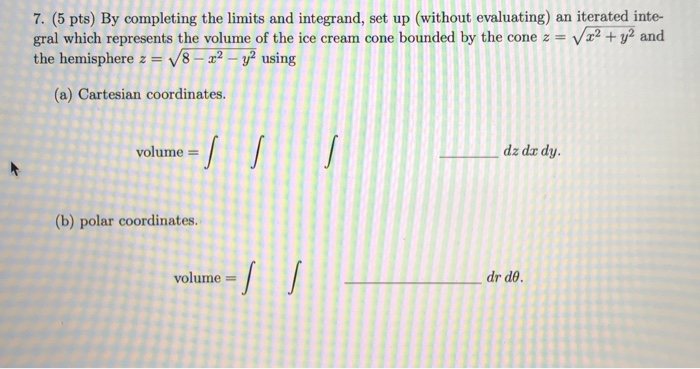7. (5 pts) By completing the limits and integrand, set up (without evaluating) an iterated inte- gral which represents the volume of the ice cream cone bounded by the cone z = V x2 + y2 and the hemisphere z = V8 - 22 - y2 using (a) Cartesian coordinates. volume = dz dar dy. (b) polar coordinates. volume = dr de.

• ### 7. (5 pts) By completing the limits and integrand, set up (without evaluating) an iterated inte-...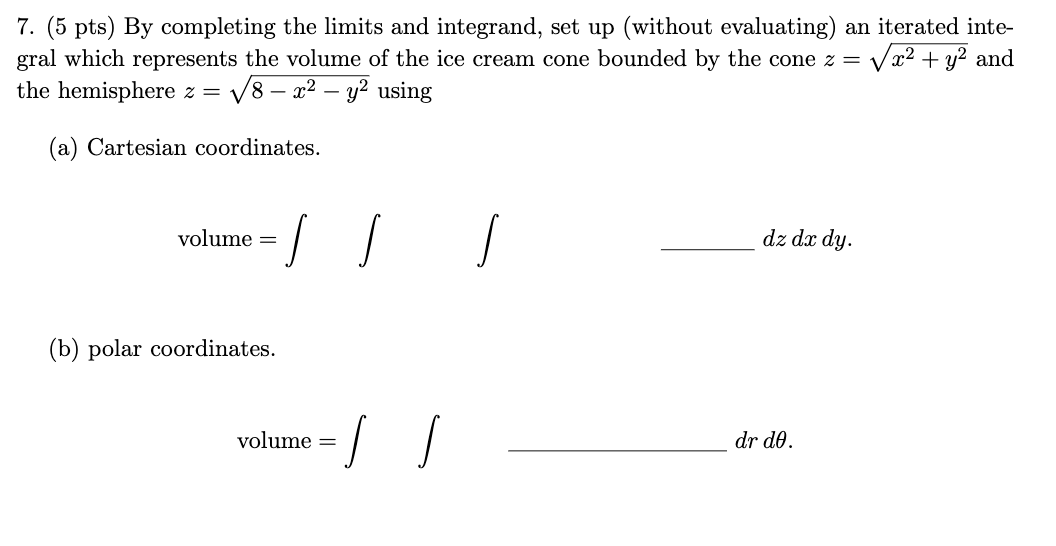7. (5 pts) By completing the limits and integrand, set up (without evaluating) an iterated inte- gral which represents the volume of the ice cream cone bounded by the cone z = V x2 + y2 and the hemisphere z = V8 – x2 - y2 using (a) Cartesian coordinates. volume = dz dx dy. (b) polar coordinates. volume = I dr de.

• ### 16. Question Details LarCalc11 14.6.017. (3865000) Set up a triple integral for the volume of the...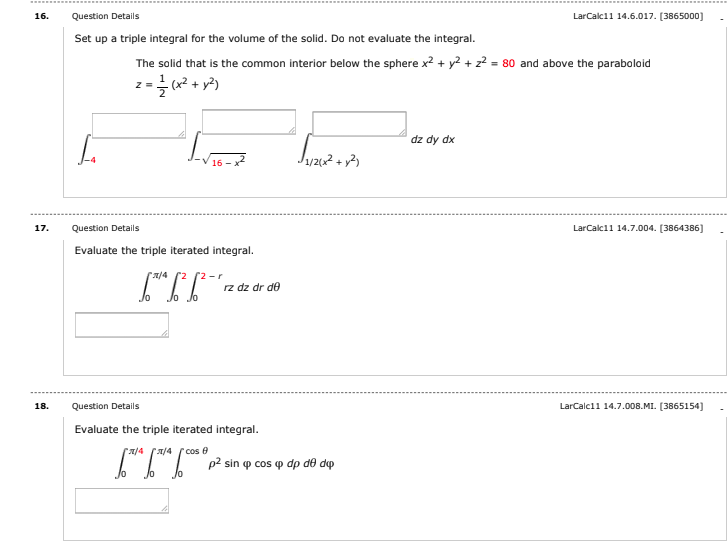16. Question Details LarCalc11 14.6.017. (3865000) Set up a triple integral for the volume of the solid. Do not evaluate the integral. The solid that is the common interior below the sphere x2 + y2 + 2+ = 80 and above the paraboloid z = {(x2 + y2) dz dy dx L J1/2012 + y2 Super 17. LarCalc11 14.7.004. (3864386] Question Details Evaluate the triple iterated integral. 6**6*6*2 2/4 2 2r rz dz dr de Jo lo 18. Question Details...

• ### Thanks In evaluating a double integral over a region D, a sum of iterated integrals was...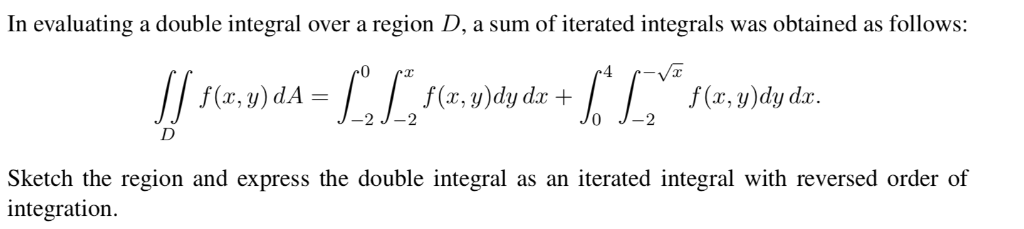Thanks In evaluating a double integral over a region D, a sum of iterated integrals was obtained as follows: 0 f(x, y)dy dr f (r, y)dy d f(x, y) dA -2 2 TJ= Sketch the region and express the double integral integration as an iterated integral with reversed order of